Here are some examples of Surface Shaders. The examples below focus on using built-in lighting models; examples on how to implement custom lighting models are in Surface Shader Lighting Examples.

## Simple

We'll start with a very simple shader and build up on that. Here's a shader that just sets surface color to "white". It uses built-in Lambert (diffuse) lighting model.

```  Shader "Example/Diffuse Simple" {
Tags { "RenderType" = "Opaque" }
CGPROGRAM
#pragma surface surf Lambert
struct Input {
float4 color : COLOR;
};
void surf (Input IN, inout SurfaceOutput o) {
o.Albedo = 1;
}
ENDCG
}
Fallback "Diffuse"
}
```

Here's how it looks like on a model with two lights set up: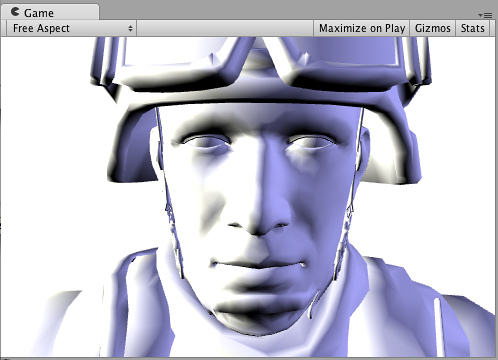## Texture

An all-white object is quite boring, so let's add a texture. We'll add a Properties block to the shader, so we get a texture selector in our Material. Other changes are in bold below.

```  Shader "Example/Diffuse Texture" {
Properties {
_MainTex ("Texture", 2D) = "white" {}
}
Tags { "RenderType" = "Opaque" }
CGPROGRAM
#pragma surface surf Lambert
struct Input {
float2 uv_MainTex;
};
sampler2D _MainTex;
void surf (Input IN, inout SurfaceOutput o) {
o.Albedo = tex2D (_MainTex, IN.uv_MainTex).rgb;
}
ENDCG
}
Fallback "Diffuse"
}
```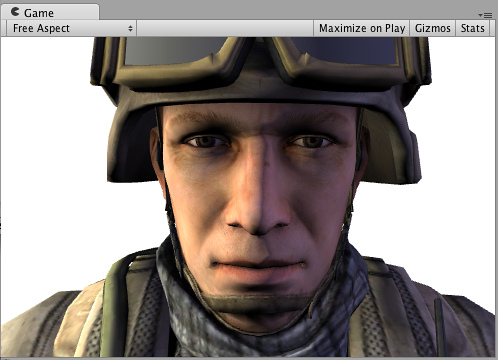## Normal mapping

```  Shader "Example/Diffuse Bump" {
Properties {
_MainTex ("Texture", 2D) = "white" {}
_BumpMap ("Bumpmap", 2D) = "bump" {}
}
Tags { "RenderType" = "Opaque" }
CGPROGRAM
#pragma surface surf Lambert
struct Input {
float2 uv_MainTex;
float2 uv_BumpMap;
};
sampler2D _MainTex;
sampler2D _BumpMap;
void surf (Input IN, inout SurfaceOutput o) {
o.Albedo = tex2D (_MainTex, IN.uv_MainTex).rgb;
o.Normal = UnpackNormal (tex2D (_BumpMap, IN.uv_BumpMap));
}
ENDCG
}
Fallback "Diffuse"
}
```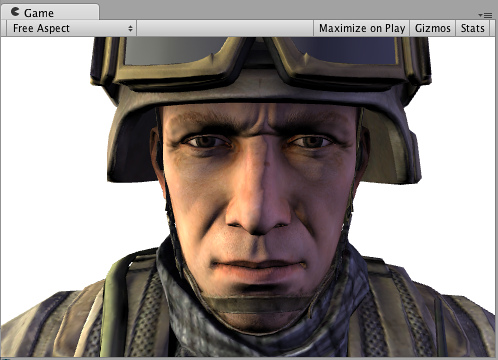## Rim Lighting

Now, try to add some Rim Lighting to highlight the edges of an object. We'll add some emissive light based on angle between surface normal and view direction. For that, we'll use `viewDir` built-in surface shader variable.

```  Shader "Example/Rim" {
Properties {
_MainTex ("Texture", 2D) = "white" {}
_BumpMap ("Bumpmap", 2D) = "bump" {}
_RimColor ("Rim Color", Color) = (0.26,0.19,0.16,0.0)
_RimPower ("Rim Power", Range(0.5,8.0)) = 3.0
}
Tags { "RenderType" = "Opaque" }
CGPROGRAM
#pragma surface surf Lambert
struct Input {
float2 uv_MainTex;
float2 uv_BumpMap;
float3 viewDir;
};
sampler2D _MainTex;
sampler2D _BumpMap;
float4 _RimColor;
float _RimPower;
void surf (Input IN, inout SurfaceOutput o) {
o.Albedo = tex2D (_MainTex, IN.uv_MainTex).rgb;
o.Normal = UnpackNormal (tex2D (_BumpMap, IN.uv_BumpMap));
half rim = 1.0 - saturate(dot (normalize(IN.viewDir), o.Normal));
o.Emission = _RimColor.rgb * pow (rim, _RimPower);
}
ENDCG
}
Fallback "Diffuse"
}
```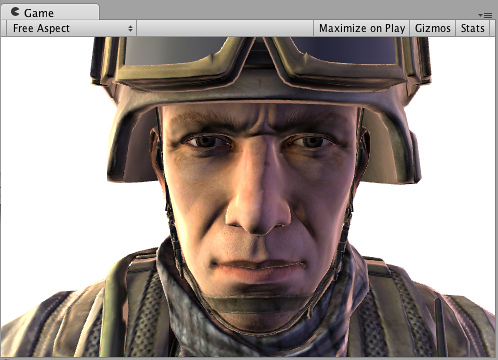## Detail Texture

For a different effect, let's add a detail texture that is combined with the base texture. Detail texture uses the same UVs, but usually different Tiling in the Material, so we have to use different input UV coordinates.

```  Shader "Example/Detail" {
Properties {
_MainTex ("Texture", 2D) = "white" {}
_BumpMap ("Bumpmap", 2D) = "bump" {}
_Detail ("Detail", 2D) = "gray" {}
}
Tags { "RenderType" = "Opaque" }
CGPROGRAM
#pragma surface surf Lambert
struct Input {
float2 uv_MainTex;
float2 uv_BumpMap;
float2 uv_Detail;
};
sampler2D _MainTex;
sampler2D _BumpMap;
sampler2D _Detail;
void surf (Input IN, inout SurfaceOutput o) {
o.Albedo = tex2D (_MainTex, IN.uv_MainTex).rgb;
o.Albedo *= tex2D (_Detail, IN.uv_Detail).rgb * 2;
o.Normal = UnpackNormal (tex2D (_BumpMap, IN.uv_BumpMap));
}
ENDCG
}
Fallback "Diffuse"
}
```

Using a checker texture does not make much practical sense, but illustrates what happens: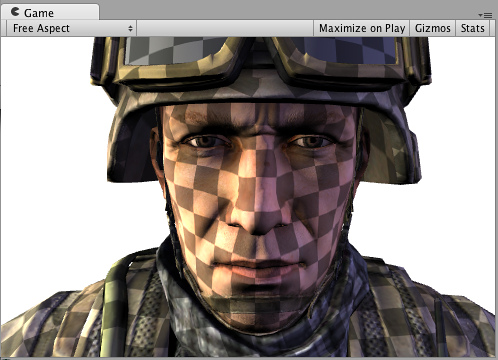## Detail Texture in Screen Space

How about a detail texture in screen space? It does not make much sense for a soldier head model, but illustrates how a built-in `screenPos` input might be used:

```  Shader "Example/ScreenPos" {
Properties {
_MainTex ("Texture", 2D) = "white" {}
_Detail ("Detail", 2D) = "gray" {}
}
Tags { "RenderType" = "Opaque" }
CGPROGRAM
#pragma surface surf Lambert
struct Input {
float2 uv_MainTex;
float4 screenPos;
};
sampler2D _MainTex;
sampler2D _Detail;
void surf (Input IN, inout SurfaceOutput o) {
o.Albedo = tex2D (_MainTex, IN.uv_MainTex).rgb;
float2 screenUV = IN.screenPos.xy / IN.screenPos.w;
screenUV *= float2(8,6);
o.Albedo *= tex2D (_Detail, screenUV).rgb * 2;
}
ENDCG
}
Fallback "Diffuse"
}
```

I removed normal mapping from the shader above, just to make it shorter: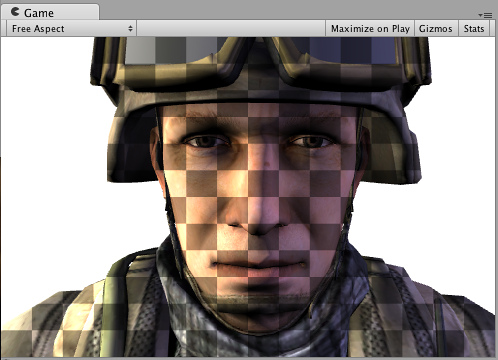## Cubemap Reflection

Here's a shader that does cubemapped reflection using built-in `worldRefl` input. It's actually very similar to built-in Reflective/Diffuse shader:

```  Shader "Example/WorldRefl" {
Properties {
_MainTex ("Texture", 2D) = "white" {}
_Cube ("Cubemap", CUBE) = "" {}
}
Tags { "RenderType" = "Opaque" }
CGPROGRAM
#pragma surface surf Lambert
struct Input {
float2 uv_MainTex;
float3 worldRefl;
};
sampler2D _MainTex;
samplerCUBE _Cube;
void surf (Input IN, inout SurfaceOutput o) {
o.Albedo = tex2D (_MainTex, IN.uv_MainTex).rgb * 0.5;
o.Emission = texCUBE (_Cube, IN.worldRefl).rgb;
}
ENDCG
}
Fallback "Diffuse"
}
```

And since it assigns the reflection color as Emission, we get a very shiny soldier: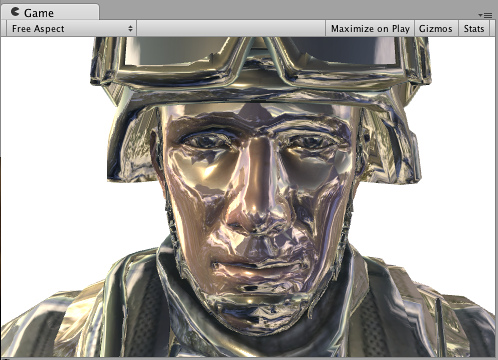If you want to do reflections that are affected by normal maps, it needs to be slightly more involved: `INTERNAL_DATA` needs to be added to the Input structure, and `WorldReflectionVector` function used to compute per-pixel reflection vector after you've written the Normal output.

```  Shader "Example/WorldRefl Normalmap" {
Properties {
_MainTex ("Texture", 2D) = "white" {}
_BumpMap ("Bumpmap", 2D) = "bump" {}
_Cube ("Cubemap", CUBE) = "" {}
}
Tags { "RenderType" = "Opaque" }
CGPROGRAM
#pragma surface surf Lambert
struct Input {
float2 uv_MainTex;
float2 uv_BumpMap;
float3 worldRefl;
INTERNAL_DATA
};
sampler2D _MainTex;
sampler2D _BumpMap;
samplerCUBE _Cube;
void surf (Input IN, inout SurfaceOutput o) {
o.Albedo = tex2D (_MainTex, IN.uv_MainTex).rgb * 0.5;
o.Normal = UnpackNormal (tex2D (_BumpMap, IN.uv_BumpMap));
o.Emission = texCUBE (_Cube, WorldReflectionVector (IN, o.Normal)).rgb;
}
ENDCG
}
Fallback "Diffuse"
}
```

Here's a normal mapped shiny soldier: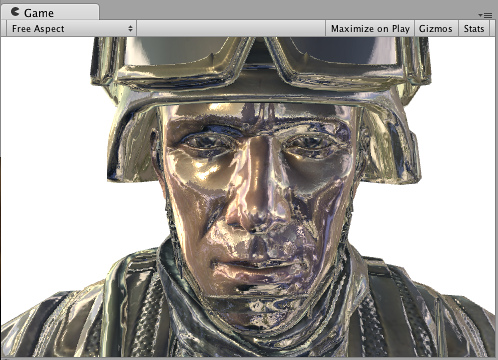## Slices via World Space Position

Here's a shader that "slices" the object by discarding pixels in nearly horizontal rings. It does that by using `clip()` Cg/HLSL function based on world position of a pixel. We'll use `worldPos` built-in surface shader variable.

```  Shader "Example/Slices" {
Properties {
_MainTex ("Texture", 2D) = "white" {}
_BumpMap ("Bumpmap", 2D) = "bump" {}
}
Tags { "RenderType" = "Opaque" }
Cull Off
CGPROGRAM
#pragma surface surf Lambert
struct Input {
float2 uv_MainTex;
float2 uv_BumpMap;
float3 worldPos;
};
sampler2D _MainTex;
sampler2D _BumpMap;
void surf (Input IN, inout SurfaceOutput o) {
clip (frac((IN.worldPos.y+IN.worldPos.z*0.1) * 5) - 0.5);
o.Albedo = tex2D (_MainTex, IN.uv_MainTex).rgb;
o.Normal = UnpackNormal (tex2D (_BumpMap, IN.uv_BumpMap));
}
ENDCG
}
Fallback "Diffuse"
}
```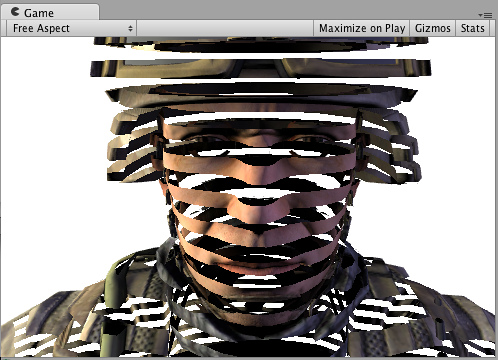## Normal Extrusion with Vertex Modifier

It is possible to use a "vertex modifier" function that will modify incoming vertex data in the vertex shader. This can be used for procedural animation, extrusion along normals and so on. Surface shader compilation directive `vertex:functionName` is used for that, with a function that takes `inout appdata_full` parameter.

Here's a shader that moves vertices along their normals by the amount specified in the material:

```  Shader "Example/Normal Extrusion" {
Properties {
_MainTex ("Texture", 2D) = "white" {}
_Amount ("Extrusion Amount", Range(-1,1)) = 0.5
}
Tags { "RenderType" = "Opaque" }
CGPROGRAM
#pragma surface surf Lambert vertex:vert
struct Input {
float2 uv_MainTex;
};
float _Amount;
void vert (inout appdata_full v) {
v.vertex.xyz += v.normal * _Amount;
}
sampler2D _MainTex;
void surf (Input IN, inout SurfaceOutput o) {
o.Albedo = tex2D (_MainTex, IN.uv_MainTex).rgb;
}
ENDCG
}
Fallback "Diffuse"
}
```

Moving vertices along their normals makes a fat soldier: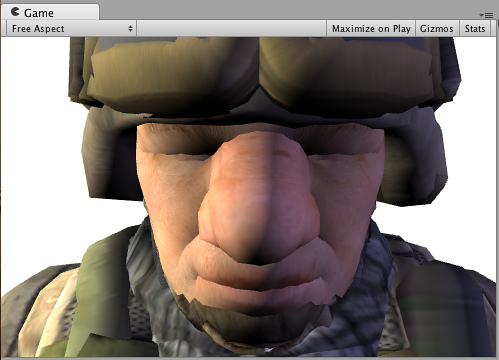## Custom data computed per-vertex

Using a vertex modifier function it is also possible to compute custom data in a vertex shader, which then will be passed to the surface shader function per-pixel. The same compilation directive `vertex:functionName` is used, but the function should take two parameters: `inout appdata_full` and `out Input`. You can fill in any Input member that is not a built-in value there.

Example below defines a custom `float3 customColor` member, which is computed in a vertex function:

```  Shader "Example/Custom Vertex Data" {
Properties {
_MainTex ("Texture", 2D) = "white" {}
}
Tags { "RenderType" = "Opaque" }
CGPROGRAM
#pragma surface surf Lambert vertex:vert
struct Input {
float2 uv_MainTex;
float3 customColor;
};
void vert (inout appdata_full v, out Input o) {
UNITY_INITIALIZE_OUTPUT(Input,o);
o.customColor = abs(v.normal);
}
sampler2D _MainTex;
void surf (Input IN, inout SurfaceOutput o) {
o.Albedo = tex2D (_MainTex, IN.uv_MainTex).rgb;
o.Albedo *= IN.customColor;
}
ENDCG
}
Fallback "Diffuse"
}
```

In this example `customColor` is set to the absolute value of the normal: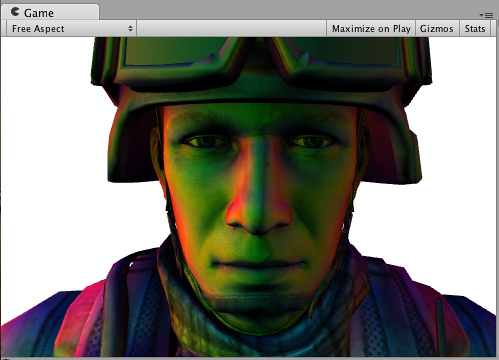More practical uses could be computing any per-vertex data that is not provided by built-in Input variables; or optimizing shader computations. For example, it's possible to compute Rim lighting at object's vertices, instead of doing that in the surface shader per-pixel.

## Final Color Modifier

It is possible to use a "final color modifier" function that will modify final color computed by the shader. Surface shader compilation directive `finalcolor:functionName` is used for that, with a function that takes `Input IN, SurfaceOutput o, inout fixed4 color` parameters.

Here's a simple shader that applies tint to final color. This is different from just applying tint to surface Albedo color: this tint will also affect any color that came from lightmaps, light probes and similar extra sources.

```  Shader "Example/Tint Final Color" {
Properties {
_MainTex ("Texture", 2D) = "white" {}
_ColorTint ("Tint", Color) = (1.0, 0.6, 0.6, 1.0)
}
Tags { "RenderType" = "Opaque" }
CGPROGRAM
#pragma surface surf Lambert finalcolor:mycolor
struct Input {
float2 uv_MainTex;
};
fixed4 _ColorTint;
void mycolor (Input IN, SurfaceOutput o, inout fixed4 color)
{
color *= _ColorTint;
}
sampler2D _MainTex;
void surf (Input IN, inout SurfaceOutput o) {
o.Albedo = tex2D (_MainTex, IN.uv_MainTex).rgb;
}
ENDCG
}
Fallback "Diffuse"
}
```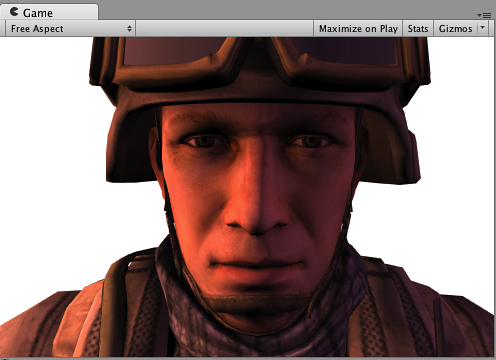## Custom Fog with Final Color Modifier

Common use case for final color modifier (see above) would be implementing completely custom Fog. Fog needs to affect the final computed pixel shader color, which is exactly what the `finalcolor` modifier does.

Here's a shader that applies fog tint based on distance from screen center. This combines both the vertex modifier with custom vertex data (`fog`) and final color modifier. When used in forward rendering additive pass, Fog needs to fade to black color, and this example handles that as well with a check for `UNITY_PASS_FORWARDADD`.

```  Shader "Example/Fog via Final Color" {
Properties {
_MainTex ("Texture", 2D) = "white" {}
_FogColor ("Fog Color", Color) = (0.3, 0.4, 0.7, 1.0)
}
Tags { "RenderType" = "Opaque" }
CGPROGRAM
#pragma surface surf Lambert finalcolor:mycolor vertex:myvert
struct Input {
float2 uv_MainTex;
half fog;
};
void myvert (inout appdata_full v, out Input data)
{
UNITY_INITIALIZE_OUTPUT(Input,data);
float4 hpos = mul (UNITY_MATRIX_MVP, v.vertex);
data.fog = min (1, dot (hpos.xy, hpos.xy) * 0.1);
}
fixed4 _FogColor;
void mycolor (Input IN, SurfaceOutput o, inout fixed4 color)
{
fixed3 fogColor = _FogColor.rgb;
fogColor = 0;
#endif
color.rgb = lerp (color.rgb, fogColor, IN.fog);
}
sampler2D _MainTex;
void surf (Input IN, inout SurfaceOutput o) {
o.Albedo = tex2D (_MainTex, IN.uv_MainTex).rgb;
}
ENDCG
}
Fallback "Diffuse"
}
```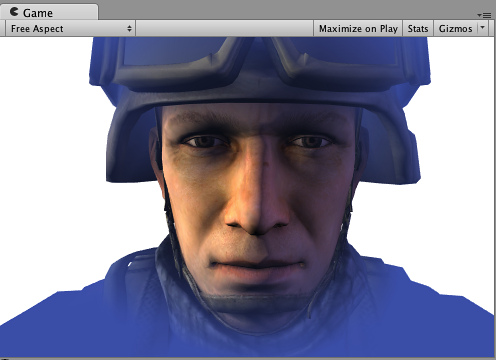## Linear Fog

```  Properties {
_MainTex ("Base (RGB)", 2D) = "white" {}
}
Tags { "RenderType"="Opaque" }
LOD 200

CGPROGRAM
#pragma surface surf Lambert finalcolor:mycolor vertex:myvert

sampler2D _MainTex;
uniform half4 unity_FogColor;
uniform half4 unity_FogStart;
uniform half4 unity_FogEnd;

struct Input {
float2 uv_MainTex;
half fog;
};

void myvert (inout appdata_full v, out Input data) {
UNITY_INITIALIZE_OUTPUT(Input,data);
float pos = length(mul (UNITY_MATRIX_MV, v.vertex).xyz);
float diff = unity_FogEnd.x - unity_FogStart.x;
float invDiff = 1.0f / diff;
data.fog = clamp ((unity_FogEnd.x - pos) * invDiff, 0.0, 1.0);
}
void mycolor (Input IN, SurfaceOutput o, inout fixed4 color) {
fixed3 fogColor = unity_FogColor.rgb;
fogColor = 0;
#endif
color.rgb = lerp (fogColor, color.rgb, IN.fog);
}

void surf (Input IN, inout SurfaceOutput o) {
half4 c = tex2D (_MainTex, IN.uv_MainTex);
o.Albedo = c.rgb;
o.Alpha = c.a;
}
ENDCG
}
FallBack "Diffuse"
```

}

Page last updated: 2013-01-15